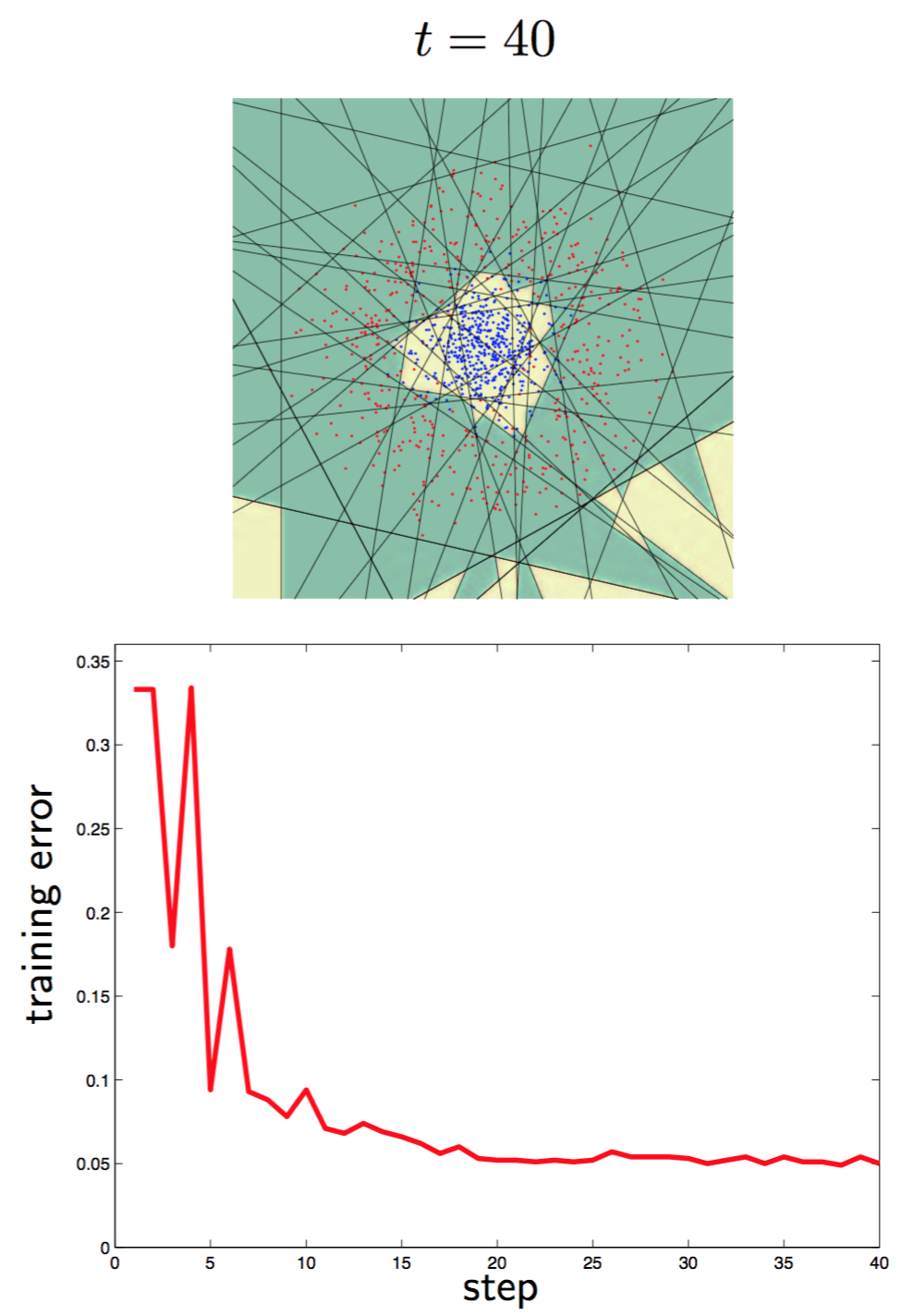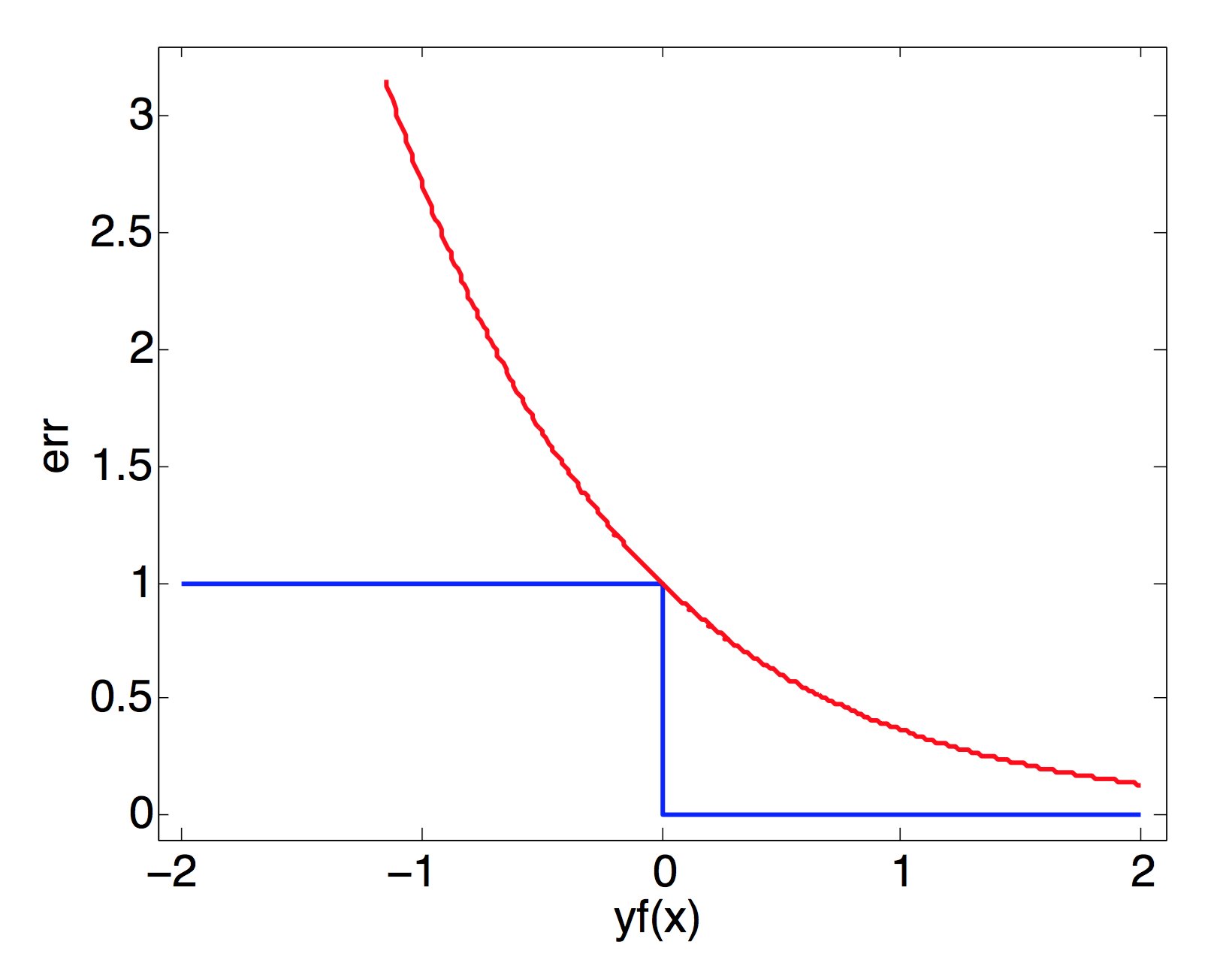The AdaBoost algorithm of Freund and Schapire was the first practical boosting algorithm, and remains one of the most widely used and studied, with applications in numerous fields.

### AlgorithmThe boosting algorithm AdaBoost

$$(x_i, y_i)$$ is a tuple where $$x_i$$ is features vector and $$y_i$$ is class label. In the begining, all tuples have same weigh represented by distribution $$D_t^i$$. In each iteration, we first train a weak learner using $$D_t$$, as long as the learner is better than random guess, the boosting can get a good result. If the learner is a decision tree, we should consider the weigh when calculating loss at every tree node for discriminator selection. By using the learner, we can get a weak hypothesis $$h_t$$ which maps features space to {-1, 1}. $$\epsilon_t$$ is the sum of weigh of misclassified tuples. By derivation, $$D_t$$ can be updated as above where $$\alpha_t$$ equals to $$\frac{1}{2}ln(\frac{1-\epsilon_t}{\epsilon_t})$$. After several rounds, we can get a strong classfier by combining trained weak learner with learner weigh $$\alpha_t$$.### Derivation by Loss Function

For simplicity we have $$H(x)=\sum_{t=1}^{T}\alpha_th_t(x)$$ Forward Stagewise Additive Modeling
Input: $$T={(x_1,y_1),(x_2,y_2),…,(x_N,y_N)}$$
Loss Func: $$L(y,f(x))$$
Base Func Set: $$b(x;\gamma)$$
Output: $$f(x)=\sum_{m=1}^{M}\beta_mb(x;\gamma_m)$$
Algorithm:

• Initial$$f_0(x)=0$$
• For m $$\in$$ {1,2,3,…,M}
• $$(\beta_m,\gamma_m)=arg\min_{\beta,\gamma}\sum_{i=1}^{N}L(y_i,f_{m-1}(x_i)+\beta b(x_i;\gamma))$$
• $$f_m(x)=f_{m-1}(x)+\beta_mb(x;\gamma_m)$$
• $$f(x)=\sum_{m=1}^{M}\beta_mb(x;\gamma_m)$$

We suppose that loss function of Adaboost is exponential loss.

$$L(y,H(x))=\exp(-yH(x))$$ $$H_t(x)=H_{t-1}(x)+\alpha_th_t(x)$$

We aim to get
$$(\alpha_t,h_t(x))=arg\min_{\alpha,h}\sum_{i=1}^{m}\exp(-y_i(H_{t-1}(x_i)+\alpha h(x_i)))$$
The solution to problem with multiple parameters, we can fix $$H_{t-1}(x_i)$$ so that $$\exp(-y_iH_{t-1}(x_i))$$ is dependent to $$\alpha\ and\ h(x_i)$$. Set

$$D_t^i=\exp(-y_iH_{t-1}(x_i))$$ $$(\alpha_t,h_t(x))=arg\min_{\alpha,h}\sum_{i=1}^{m}D_t^i\exp(-y_i\alpha h(x_i))$$

For solving this equation, $$h_t(x)$$ has another equation.

$$h_t^*(x)=arg\min_h\sum_{i=1}^{m}D_t^iI(y_i \ne h(x_i))$$

We can solve this by training a weak model.
Consider $$y_i \in \{-1,+1\}$$

$$\sum_{i=1}^{m}D_t^i\exp(-y_i\alpha h(x_i))$$ $$=\sum_{y_i=h_t(x_i)}D_t^ie^{-\alpha}+\sum_{y_i \ne h_t(x_i)}D_t^ie^{\alpha}$$ $$=\sum_{y_i=h_t(x_i)}D_t^ie^{-\alpha}+\sum_{y_i \ne h_t(x_i)}D_t^ie^{\alpha}$$ $$=e^{-\alpha}\sum_{i=1}^{m}D_t^i + (e^{\alpha}-e^{-\alpha})\sum_{i=1}^{m}D_t^iI(y_i \ne h(x_i))$$

From equation above, we can say that $$h_t^{*}(x)$$ can replace $$h_t(x_i)$$
Next, we need to get $$\alpha_t$$
$$\alpha_t=arg\min_{\alpha}e^{-\alpha}\sum_{i=1}^{m}D_t^i + (e^{\alpha}-e^{-\alpha})\sum_{i=1}^{m}D_t^iI(y_i \ne h_t^*(x_i))$$
Calculate derivative of the right part and make it 0 to get $$\alpha_t^*$$
We can get

$$\alpha_t^*=\frac{1}{2}ln\frac{1-e_t}{e_t}$$ $$e_t=\sum_{i=1}{m}D_t^iI(y_i \ne h_t^*(x_i))$$ $$\downarrow$$ $$e_t == \epsilon_t$$ $$D_{t+1}^i=\exp(-y_i(f_{m-1}(x_i)+\alpha_th_t(x_i)))=D_t^i\exp(-y_i\alpha_th_t(x_i))$$

This equation is similar to the update equation in Adaboost except for the normalization coefficient $$Z_t$$.

### Derivation by Upper Bound

#### Theorem:

Error is minimized by minimizing $$Z_t$$

#### Proof:

$$D_{t+1}^i=\frac{1}{m} \cdot \frac{e^{-y_i\alpha_1h_1(x_i)}}{Z_1} \cdot \ldots \cdot \frac{e^{-y_i\alpha_Th_T(x_i)}}{Z_T}$$ $$=\frac{e^{\sum_{t=1}^{T}-y_i\alpha_th_t(x_i)}}{m\prod_{t=1}^TZ_t}$$ $$=\frac{e^{-y_i\sum_{t=1}^{T}\alpha_th_t(x_i)}}{m\prod_{t=1}^TZ_t}$$ $$=\frac{e^{-y_if(x_i)}}{m\prod_{t=1}^TZ_t}$$

From figure below, we can find that $$I(H(x_i) \ne y_i) \le e^{-y_if(x_i)}$$Thus we have

$$\frac{1}{m}\sum_{i=1}^{m}I(H(x_i) \ne y_i) \le \frac{1}{m}\sum_{i=1}^{m}e^{-y_if(x_i)}$$ $$=\sum_{i=1}^{m}(\prod_{t=1}^{T}Z_t)D_{t+1}^i$$ $$=\prod_{t=1}^{T}Z_t$$ Because $$\sum_{i=1}^{m}D_{t+1}^i == 1$$

According to this theorem, we should choose $$\alpha_t$$ to minimize $$Z_t$$
Remember that $$Z_t$$ is the normalization factor, so $$Z_t=\min_{\alpha_t}\sum_{i=1}^{m}D_t^i\exp(-y_i\alpha_th_t(x_i))$$
Making the first derivative of $$Z_t$$ with respect to $$\alpha_t$$ equals to zero

$$\frac{\delta Z_t}{\delta \alpha_t}=-\sum_{i=1}^{m}D_t^iy_ih_t(x_i)e^{-y_i\alpha_th_t(x_i)}=0$$ $$-\sum_{y_i=h_t(x_i)}D_t^ie^{-\alpha_t} + \sum_{y_i \ne h_t(x_i)}D_t^ie^{\alpha_t}=0$$ $$-(1-\epsilon_t)e^{-\alpha_t} + \epsilon_te^{\alpha_t}=0$$ $$\alpha_t=\frac{1}{2}ln\frac{1-\epsilon_t}{\epsilon_t}$$

The result is the same as deviations above.